# Real-Time High Performance of Induction Motor Drive Using Hybrid Fuzzy-Sliding Mode Controllers

Real-Time High Performance of Induction Motor Drive Using Hybrid Fuzzy-Sliding Mode Controllers

Amar Bouayad DebbaghMokhtar Bendjebbar Mohamed Benslimane Mokhtar Zerikat Ahmed Allali

Laboratoire d’Automatique et d’Analyse des Systèmes (LAAS), Département de Génie Electrique, Ecole Nationale Polytechnique d’Oran Maurice Audin, Oran 31000, Algeria

Diagnostic Group LDEE, Université des Sciences et de la Technologie M.B., Oran 31000, Algeria

Département d’Electrotechnique, LDDEE Laboratory, Université des Sciences et de la Technologie d’Oran M.B., Oran 31000, Algeria

Corresponding Author Email:
Page:
903-908
|
DOI:
https://doi.org/10.18280/jesa.540613
30 October 2021
|
Accepted:
22 December 2021
|
Published:
29 December 2021
| Citation

OPEN ACCESS

Abstract:

Obtaining the required performance, stability, and robustness in real-time control of induction motors usually requires the use of complex controllers, however through multiple experimentations, many challenges have arisen from such methods.  The complex structure of control methods in real-time applications is usually computationally challenging and energy consuming, hence the need for a simple control strategy to overcome these challenges, in this paper, we focus on designing an advanced hybrid control strategy with a simple design applied to an induction motor. Mainly, the hybrid controller used in this study has the benefits of joining the best performance of both fuzzy logic controller and sliding mode controller, specifically designed to handle each phase separately, the transition phase and the steady phase. A fuzzy controller intervenes as a supervisor in our control structure, more specifically it manages the switch from one type of control to the other taking into account the intervention phase of each type of controller by commanding the rate of both controllers. Control performance analysis was carried out in a real experimental setup to validate the efficiency and robustness of the proposed hybrid controller and confirm its effectiveness in handling the compromise between overshoot and response time.

Keywords:

Dspace, fuzzy logic control, induction motor, real-time implementation, robustness, sliding mode control, supervisor

1. Introduction

The application of indirect flux-oriented control (IFOC) for induction motors (MI) has been widely used to ensure decoupling between torque and flux, thus obtaining a model similar to that of a current machine continuous . In this case, different conventional controllers can be used to ensure the desired performance. However, certain performances cannot be guaranteed in the presence of structural variations or external disturbances. It is then necessary to synthesize robust commands regarding these disturbances. In this paper we opted for a hybrid approach by combining two robust control laws: sliding mode control (SMC) and fuzzy control (FLC).

Although the SMC proved many times its robustness and effectiveness against structural uncertainties and external disturbances [2, 3]. SMC presents a grand factor for initiating the chattering phenomenon that can damage the system at high frequencies.

To remedy this inconvenience, several solutions have been proposed in the literature. Some authors introduced a transition band around the sliding surface [3, 4]. However, a compromise must be found between the tracking performance and the width of this band. Others introduced an integrator block at the output of the controller. In this case, the chatter phenomenon is certainly reduced [5, 6], but the tracking error persists.

In order to keep the robustness of the sliding mode and eliminate the phenomenon of chattering, without deteriorating the performance of the system, several “hybrid” approaches have been developed [7-9]. These approaches are used to obtain the best performances by combing different control methods. The proposed method to get over the chattering caused by the sliding mode control is a fuzzy adaptive PI-sliding mode control . In order to enhance the combination of the FLC and SMC they use genetic algorithms, and thus minimize the chattering phenomenon . The authors  combine a sliding mode command and another of the H∞ type to improve the tracking performance of their system.

In this paper, we rely on a fuzzy logic supervisor to allow gradual switching between two control laws: sliding mode control which is used mainly during the transient phase, and a fuzzy logic controller that will take over during the steady phase, the fuzzy logic controller is mainly used for its ability to enhance performance and guarantee total reject of chattering. Sliding mode control is known for its challenging implementation procedure due to the violent chattering that may result from such method, using a specific hybrid form which involves fuzzy supervisor, the main contribution of the proposed scheme is to simplify the combination of the first-order sliding mode control and a fuzzy logic controller. to assess the effectiveness of the proposed strategy on an induction machine, a real-time comparative study is proposed in section 6.

2. Induction Machine Model

To control the induction machine, we opted for a voltage control, by using the current components (isd, isq) and flux components (φrd, φrq) as control variables and state variable respectively we obtain the below mathematical model that represent the reduced model linked to stator reference.

$\frac{d \omega_{r}}{d t}=\frac{n_{p} M}{J L_{r}} \cdot\left(\varphi_{r d} i_{s q}+\varphi_{r q} i_{s d}\right)-\frac{T_{L}}{J}$           (1)

$\frac{d i_{S d}}{d t}=\frac{R_{r} M}{\sigma L_{s} L_{r}^{2}} \cdot \varphi_{r d}+\frac{n_{p} M}{\sigma L_{s} L_{r}} \cdot \varphi_{r d} \cdot \omega_{r}-\frac{R_{r} M^{2}+L_{r}^{2}}{\sigma L_{s} L_{r}^{2}} \cdot i_{s d}$                 (2)

$\frac{d i_{s q}}{d t}=\frac{R_{r} M}{\sigma L_{s} L_{r}^{2}} \cdot \varphi_{r q}-\frac{n_{p} M}{\sigma L_{s} L_{r}} \cdot \varphi_{r d} \cdot \omega_{r}-\frac{R_{r} M^{2}+L_{r}^{2}}{\sigma L_{s} L_{r}^{2}} \cdot i_{s q}+\frac{1}{\sigma L_{s}} . U_{s q}$            (3)

$\frac{d \varphi_{r d}}{d t}=-\frac{R_{r}}{L_{r}} \cdot \varphi_{r d}-n_{p} \omega_{r} \varphi_{r q}+\frac{R_{r}}{L_{r}} M i_{s d}$            (4)

$\frac{d \varphi_{r q}}{d t}=-\frac{R_{r}}{L_{r}} \cdot \varphi_{r q}+n_{p} \omega_{r} \varphi_{r d}+\frac{R_{r}}{L_{r}} M i_{s q}$          (5)

where,

$\sigma=1-\frac{M^{2}}{L_{s} L_{r}}$

The electromagnetic torque is found as:

$C_{e}=\frac{2 p M}{3 L_{r}} \cdot\left(\varphi_{r d} i_{s q}+\varphi_{r q} i_{s d}\right)$         (6)

The considered approach is to drive the IM as a DC rotor (separated excitation), using the IFOC (the indirect field-oriented control), the approach is based on transforming and simplifying the 3 phased dynamic model to a system representation of 2 axes (d,q), its principal compromises on dropping the flux’s quadratic component $\varphi_{r q}$, and to keep direct variable $\varphi_{r d}$ .

$\varphi_{r q}=0 \rightarrow \varphi_{r}=\varphi_{r d}$          (7)

The above step will allow the flux control by the current $i_{s d}$  and the torque by the current $i_{s q}$ separately, yet we need to determine the amplitude and torque’s filed position.

The amplitude is determined through a nonlinear defluxing function delivered by the relation:

$\varphi_{r d}^{*}=\left\{\begin{array}{lll}\varphi_{r} & \text { if } & |\omega| \leq \omega_{n} \\ \varphi_{r n} \cdot \frac{\omega_{n}}{|\omega|} & \text { if } & |\omega|>\omega_{n}\end{array}\right.$                   (8)

The position is obtained by the stator pulsation integration, that is reconstituted from the rotor pulsation and the rotor speed.

$\theta_{s}=\int \omega_{s} \cdot d t=\int\left(p \cdot \omega+\frac{L_{m} i_{s q}}{T_{r} \varphi_{r q}}\right) \cdot d t$         (9)

3. Sliding Mode Control (SMC)

Sliding mode control is a nonlinear control method that alters the dynamics of a nonlinear system by application of a discontinuous control signal that forces the system to "slide" along a cross-section (called sliding surface) of the system's normal behavior. A general equation form has been proposed by Slotine to define the sliding surface S(x) that guarantee the convergence towards defined path .

$S(x)=\left(\frac{\partial}{\partial t}+\lambda\right)^{n-1} e(x)$         (10)

where, e(x): error; λ: positive constant; n: system order.

The objective of the controller is to maintain the surface S(x)=0. With an appropriate choice of the parameter $\lambda$, the unique solution for this differential equation is e(x)=0.

This drives to a pursuing problem corresponding to an exact linearization of the deviation e(x) while respecting the convergence condition defined by Lyapunov .

As the Lyapunov function is a scalar function V(x)> 0 for the system states, the controller must decrease this function: $\dot{V}(x)<0$, where $\dot{V}(x)$  is V(x) derivative.

The idea is to choose a scalar function S(x) to guarantee the controlled variable drive towards its reference value and to design a command u (t) such that the square of the surface corresponds to a Lyapunov function.

$V(x)=\frac{1}{2} S^{2}(x)>0$

and its derivative,

$S(x) \dot{S}(x)<0$

where, $\dot{S}(x)$ is $S(x)$ derivative.

The sliding mode exists when switching takes place continuously between ‘Umax’ and ‘Umin’, as shown in Figure 1.

## 1.png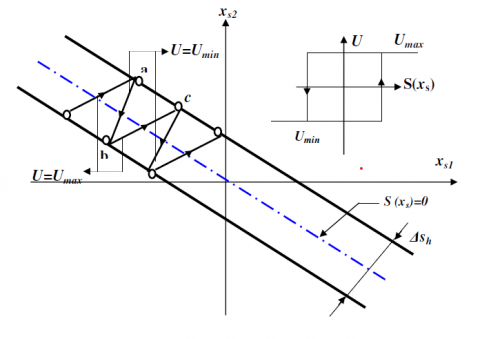Figure 1. Sliding mode principle

An ideal sliding mode is a command that switches to an infinite frequency where there is no controller that can perform this operation. Despite the various advantages of sliding mode control, its use has been impeded by a major inconvenience related to the phenomenon of chattering.

This phenomenon is a natural consequence of the actual dynamic behavior of the whole system to be controlled. the chatter can cause early deterioration of the controller or excite high dynamic frequencies not considered in the modeling of the system.

Different methods to limit this phenomenon have been developed. One approach is to replace the sign function with a smoother function, another using superior order sliding mode, others with the twist or supertwist . in this work we will use the hybrid approach with a fuzzy logic controller applied at chattering phase generally that occurred in the steady phase.

4. Fuzzy Logic Control

The fuzzy logic consists of adapting the linguistic information that emerge from human experience in order to describe system’s dynamic behavior around known operating points. This information is described by a group of rules (If-Then) [12, 13]. Regarding the induction machine, the FLC is constitute from two variables e, $\dot{e}$, the error and its derivative respectivly as the inputs, and the command UFLC as output.

More in details the fuzzy logic control is a system constituted of blocks that adapt the inputs signal to a fuzzy language, treat the fuzzy signal and adapt the output to a non-fuzzy signal, as demonstrated in Figure 2.

## 2.pngFigure 2. FLC structure

4.1 Fuzzification

The fuzzification is a process that define the membership degree of the entries using the membership functions. Figure 3 presents the error between the output and its reference and error’s derivative, respectively $e, \dot{e}$ (the inputs), the command UFLC (the output) using the membership functions. The membership functions are represented in standardized speech universe between [-1,1] . The trapezoidal and triangular forms are used to define the inputs membership functions, and the singleton form for the output membership function.

The inputs are given as the below:

$e(k)=\omega(k)_{r e f}-\omega(k)_{r}$

$d e(k)=e(k)-e(k-1)$           (11)

e(k), de(k) represent the error and its derivative in discrete time.

4.2 Rules and inferences

The inference rules define the conduct of the fuzzy regulator. It should in this manner incorporate intermediate steps that permit to pass from values to fuzzy values and vice versa; these are the fuzzification and defuzzification phases.

## 3.png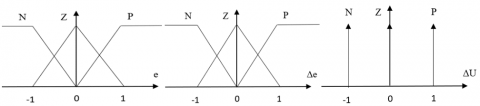Figure 3. Membership function

To synthesize the fuzzy controller, we have divided the error speech universe and its derivative into three sets: N, Z, P. Thus, using all the possible combinations, 9 fuzzy rules were generated for three singletons at the level of the consequence part as shown in Table 1.

We applied a matrix of 3x3 that constitute a table of 9 rules, with just 3 subsets membership functions (Figure 3) to enhance and decrease the calculation time, since we are restricted by the Dspace processor.

Table 1. Fuzzy logic control inference rules

 e $\Delta e$ N Z P N N N Z Z N Z P P Z P P

4.3 Defuzzification

Last phase consists into covert the fuzzy output valor using the defuzzification methods (center gravity method in our case) in order to obtain a real value that can command our machine. As shown in Figure 4, the FLC structure applied on the IM.

## 4.png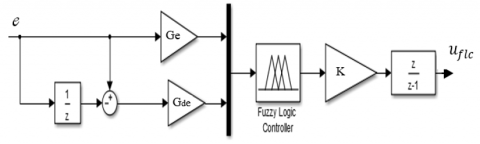Figure 4. FLC structure for IM speed control

5. Hybrid Control

In order to guarantee a quick response, stable and robust system states with the finest possible performances, we opted for a combination of both fuzzy logic and sliding mode controllers defined above, where the SMC intervenes in the transition phase and the FLC during the steady state. The first controller establishes the convergence of the response to its permanent regime with insensibility to structural and external disturbances and rapid dynamics. During which the second controller ensures the steady phase to provide smoother control and practically zero static error [8, 10].

To prevent a brusque switch between the two controllers, a progressive transition is solicited with the following form:

$U=\alpha U_{F L C}+(1-\alpha) U_{S M C}$        (12)

A fuzzy supervisor generates the variable ‘α’ that represent a weighting factor that interfere in (12), for that the supervisor need the tracking error and its derivation as inputs.

The supervisor mechanism is such α takes the value ‘0’ and ‘1’ (gradually) depending on the system state, more the tracking error and its derivatives converge to zero more the output ‘α’ converge toward the value ‘1’ .

Table 2. Supervisor FLC rules

 de/e Z M H Z TH B M M M Z Z H Z Z Z

Thus, to reduce the calculation time and simplify the structure of the supervisor, only the absolute values of the inputs have been considered. This reduces the rules to only nine components as shown in Table 2.

The structure of the proposed approach is illustrated by the diagram in Figure 5. The three blocks SMC, FLC and the fuzzy supervisor have as inputs the speed error and its derivative and USMC, UFLC, α as their respective outputs.

## 5.pngFigure 5. Hybrid controller structure

6. Experimental Results

To validate our approach, using a DSpace card 1104 and a test bench (Figure 6) we present in the following experiment results of an induction machine whose parameters are presented in Table 3.

In order to prove the superiority and advantage of the Hybrid approach compared to FLC and sliding mode controller we applied a simple benchmark on the three controllers, FLC, SMC and Hybrid Fuzzy-sliding mode controller as shown in Figure 7, with an external disturbance (15 N.m) applied on the three controllers at 7.4s.

Table 3. Induction motor parameters

 Induction Motor parameters UN 380 V PN 3 kW p 2 nN 1410 tr/min Rr 2.8 ohm Rs 6 ohm Lr 0.5142 H Ls 0.5668 H J 0.058 m². kg Msr 0.5142 H f 0.005

## 6.png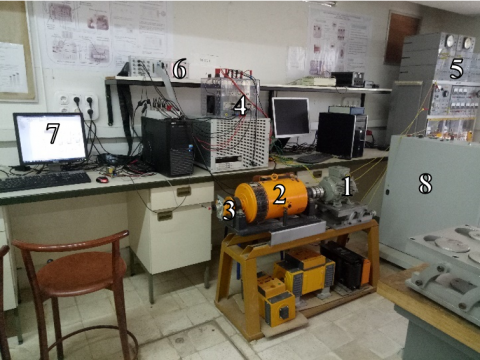1 Induction motor 5 Loads 2 Generator 6 Dspace card 3 Encoder 7 PC 4 Inverter 8 Voltage supply

Figure 6. Test bench

Figures 7-10 show the results obtained from each controller. In Figure 7 we can observe the overview of the desired speed schema with the reach of the three controllers types the reference all along the test where we can notice mainly the chattering on the SMC and the overshoots on the FLC. A zoom (Figure 8) shows that the hybrid control presents the best compromise between overshoot, response time and settling time, a quantitative analysis is presented in Table 4.

Table 4. Quantitative comparison

 SMC FLC Hybrid Rise time (s) 0.395 0.293 0.298 Overshoot 0% 13.8% 2.9% Settling time (s) 0.963 1.295 0.816

In order to test the controller’s efficiency against disturbances we applied a charge at t=7.4s on the three controllers, Figure 9 show the response accuracy of the machine with each controller, a quantitative analysis is presented in Table 5.

Figure 10 represent the speed change and its inversion, with the disturbance applied all along the test. the three controllers follow the reference with different performances, once again the hybrid command confirmed its efficiency.

Table 5. Quantitative comparaison (Disturbance case)

 SMC FLC Hybrid Time response at 1% 0.49 0.82 0.61 Average static error (rad/s) 1.9 0.51 0.55

Running the machine for a long period of time will heat the machine and consequently affect its parameters, we had to undergo the experiment by running the machine for several (hours) and test the system robustness with each controller, we can see in Figures 7-10 how the performance of the hybrid controller surpasses the other two.

## 7.png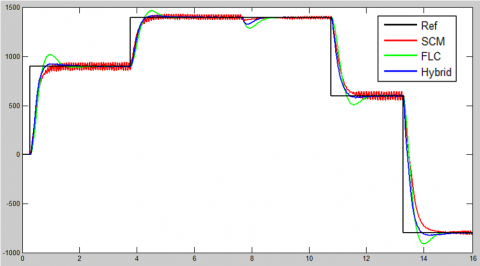Figure 7. Rotor speed regulation (rpm/sec)

## 8.png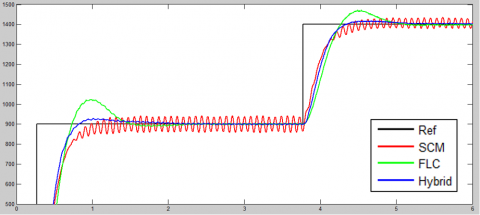Figure 8. Rotor speed regulation (transition phase) (rpm/sec)

## 9.png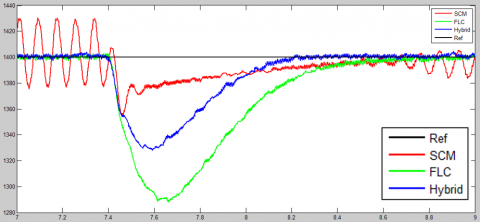Figure 9. Speed plot at the disturbance. (rpm/sec)

## 10.png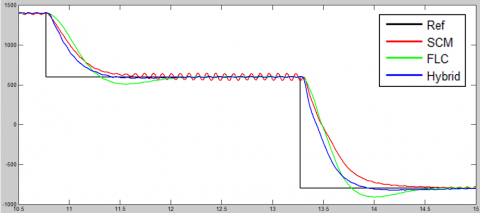Figure 10. Speed plot during the disturbance with speed changes. (rpm/sec)

## 11.png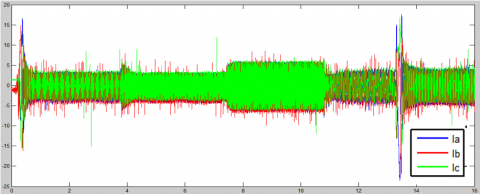Figure 11. Currents Measurement. (ampere/ sec)

## 12.png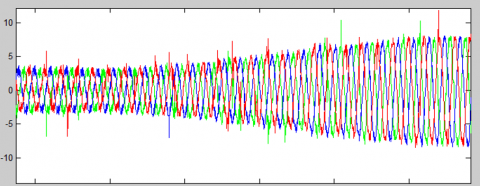Figure 12. Currents plot. (ampere/ sec)

## 13.pngFigure 13. Current plot at load application. (ampere/ sec)

Figure 11 presents the form of the hybrid controller’s currents in respect with the reference schema applied, results that comply with the theory studied above. Where currents variates in accordance with the speed plot, and attain “In” at the charge application as presented in Figure 12.

Figure 13 is a zoom on hybrid controller’s currents after disturbance application, that served as harmonic filter and give to the currents a clear and balanced sinusoidal form.

7. Conclusions

In this paper, a hybrid control of an induction machine was presented. The use of a supervisor based on a fuzzy logic ensures a gradual switching between a sliding mode controller and a fuzzy logic controller when approaching steady state. The purpose of this structure is to exploit the robustness and speed of the sliding mode during the transient state and the flexibility of the fuzzy controller during the steady state. Several experimental and comparative results were presented to validate the proposed approach. The tests results affirm he hypothesis and the efficiency of the hybrid approach compared to the classic regulators strategies, furthermore the machine can be impacted by the test environment and bias its parameters once again the strategies demonstrate its robustness against those changes.

For future work we plan to use the same approach with higher order sliding modes combined with sliding mode observers in order to ensure finite-time convergence and to improve performance.

Nomenclature
 np Number of pairs poles M Mutual inductance, H Rr Rotor resistance, Ohm Rs Stator resistance, Ohm Lr Rotor self-inductances, H Ls Stator self-inductances, H σ dispersion coefficient J Inertia moment, Kg.m2 f Friction Coefficient, Nm/rad/s TL Torque load, Nm Ref Reference, desired speed IM Induction machine
References

 Yousef, A., Abdelmaksoud, S.M. (2015). Review on field oriented control of induction motor. Int. J. Res. Emerg. Sci. Technol. (IJREST), 2(7): 5-16.

 Panchade, V.M., Chile, R.H., Patre, B.M. (2013). A survey on sliding mode control strategies for induction motors. Annual Reviews in Control, 37(2): 289-307. https://doi.org/10.1016/j.arcontrol.2013.09.008

 Jamoussi, K., Ouali, M., Charradi, H. (2007). A sliding mode speed control of an induction motor. American Journal of Applied Sciences, 4(12): 987-994. https://doi.org/10.3844/ajassp.2007.987.994

 Slotine, J.J.E., Li, W. (1991). Applied Nonlinear Control. Englewood Cliffs, NJ: Prentice Hall.

 Mahieddine-Mahmoud, S., Chrifi-Alaoui, L., Pinchon, D., Bussy, P. (2006). Robust sliding mode non linear control using integrator corrector for an induction motor. In Proceedings of the 45th IEEE Conference on Decision and Control, pp. 1623-1628. https://doi.org/10.1109/CDC.2006.377093

 Ho, E.Y., Sen, P.C. (1991). Control dynamics of speed drive systems using sliding mode controllers with integral compensation. IEEE Transactions on Industry Applications, 27(5): 883-892. https://doi.org/10.1109/28.90343

 Habbab, M., Hazzab, A., Sicard, P. (2018). Real time implementation of fuzzy adaptive pi-sliding mode controller for induction machine control. International Journal of Electrical and Computer Engineering (IJECE), 8(5): 2883-2893. https://doi.org/10.11591/ijece.v8i5.pp2883-2893

 Lin, S.C., Chen, Y.Y. (1995). A GA-based fuzzy controller with sliding mode. In Proceedings of 1995 IEEE International Conference on Fuzzy Systems, 3: 1103-1110. https://doi.org/10.1109/FUZZY.1995.409821

 Hamzaoui, A., Manamanni, N., Essounbouli, N., Zaytoon, J. (2003). Switching controllers' synthesis: combination of a sliding mode and H infinity control by a fuzzy supervisor. IFAC Proceedings Volumes, 36(6): 247-252. https://doi.org/10.1016/S1474-6670(17)36439-X

 Benharir, N., Zerikat, M., Chekroun, S., Mechernene, A. (2012). Design and analysis of a new fuzzy sliding mode observer for speed sensorless control of induction motor drive. International Review of Electrical Engineering-Iree, 7(5): 5557-5565. https://doi.org/10.1109/ICMIC.2016.7804133

 Ammar, A., Bourek, A., Benakcha, A. (2016). Implementation of robust SVM-DTC for induction motor drive using second order sliding mode control. In 2016 8th International Conference on Modelling, Identification and Control (ICMIC), pp. 338-343. https://doi.org/10.1109/ICMIC.2016.7804133

 Mechernene, A., Zerikat, M., Hachblef, M. (2008). Fuzzy speed regulation for induction motor associated with field-oriented control. IJ-STA, 2(2): 804-817.

 Zerikat, M., Bendjebbar, M., Benouzza, N. (2005). Dynamic fuzzy-neural network controller for induction motor drive. World Academy of Science, Engineering and Technology, 1(10): 278-283. https://doi.org/10.5281/zenodo.1079534